NEET  >  NEET Previous Year Questions (2014-22): Nuclei

# NEET Previous Year Questions (2014-22): Nuclei - Notes | Study Physics Class 12 - NEET

 1 Crore+ students have signed up on EduRev. Have you?

Q.1. In the given nuclear reaction, the element X is      (2022)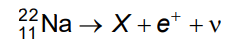(1)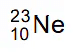(2)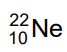(3)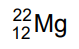(4)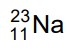Ans: (2)
Solution: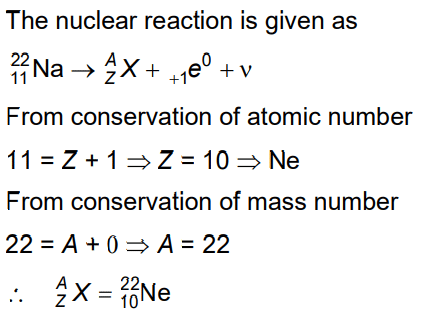Q.2. A nucleus with mass number 240 breaks into fragments each of mass number 120, the binding energy per nucleon of unfragmented nuclei is 7.6 MeV while that of fragments is 8.5 MeV. The total gain in the Binding Energy in the process is:    (2021)
A: 804 MeV
B: 216 MeV
C: 0.9 MeV
D: 9.4 MeV
Ans:
B
Solution:Given binding energy per nucleon of X, Y & Z are 7.6 MeV, 8.5 MeV & 8.5 MeV respectively.
Gain in binding energy is :-
Q = Binding Energy of products – Binding energy of reactants
= (120 × 8.5 × 2) – (240 × 7.6) MeV
= 216 MeV

Q.3. A radioactive nucleus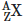undergoes spontaneous decay in the sequenceWhere Z is the atomic number of element X. The possible decay particles in the sequence are :     (2021)
A:  β+,α,β-
B: β-,α,β+

C: α,β-+
D: α,β+-
Ans:
A
Solution:β+ decreases atomic number by 1
α decreases atomic number by 2
β- decreases atomic number by 1

Q.4. The half-life of a radioactive nuclide is 100 hours. The fraction of original activity that will remain after 150 hours would be:     (2021)
A: 2/3
B: 2/3√2
C: 1/2
D: 1/2√2

Ans: D
Solution: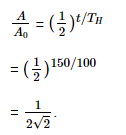Q.5. The energy equivalent of 0.5 g of a substance is :     (2020)
A: 1.5×1013 J
B: 0.5×1013J
C: 4.5×1016J
D: 4.5×1013J

Ans: D
Solution:
E = Δmc2 = 0.5 × 10-3 × (3 × 108)2
= 0.5 ×10-3 × 9 ×1016
E = 4.5 ×1013 J

Q.6. When a uranium isotope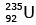is bombarded with a neutron, it generates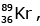three neutrons and :      (2020)
A: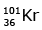B: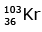C: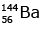D: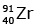Ans: C
Solution: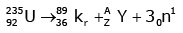92 = 36 + Z; ← A + 89 + 3 = 235 ⇒ A = 144
Z = 56

Q.7. For a radioactive material, half-life is 10 minutes. If initially there are 600 number of nuclei, the time taken (in minutes) for the disintegration of 450 nuclei is:    (2018)
A: 20
B: 10
C: 30
D: 15
Ans:
A
Solution: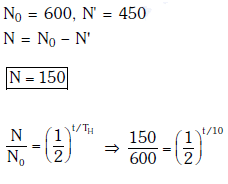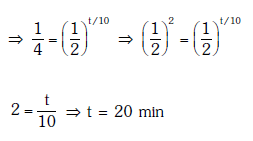Q.8. Radioactive material 'A' has decay constant '8 λ' and material 'B' has decay constant 'λ'. Initially they have same number of nuclei. After what time, the ratio of number of nuclei of material 'B' to that 'A' will be 1/e?     (2017)
A: 1/7λ
B: 1/8λ
C: 1/9λ
D: 1/10λ
Ans: A
Solution: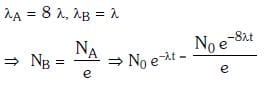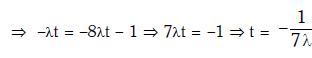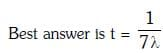Q.9. The Binding energy per nucleon of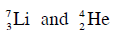nuclei are 5.60 MeV and 7.06 MeV, respectively. In the nuclear reaction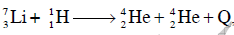the value of energy Q released is :    (2014)
A: 8.4 MeV
B: 17.3 MeV
C: 19.6 MeV
D: −2.4 MeV
Ans: B
Solution: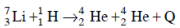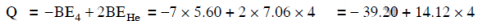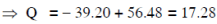Q.10. A radio isotope ‘X’ with a half life 1.4 × 109 years decays to ‘Y’ which is stable. A sample of the rock from a cave was found to contain ‘X’ and ‘Y’ in the ratio 1 : 7. The age of the rock is    (2014)
A: 4.20 × 109 years
B: 8.40 × 109 years
C: 1.96 × 109 years
D: 3.92 × 109 years
Ans: A
Solution:

Ratio of X:Y is given = 1:7
That is,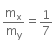⇒ 7mx = my
Let, the initial total mass is m.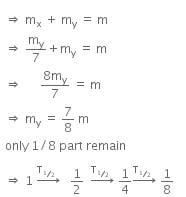Therefore, time taken to become 1/8 unstable part
= 3 x T1/2
= 3 x 1.4 x 109
= 4.2 x 109 y

The document NEET Previous Year Questions (2014-22): Nuclei - Notes | Study Physics Class 12 - NEET is a part of the NEET Course Physics Class 12.
All you need of NEET at this link: NEET

## Physics Class 12

157 videos|452 docs|213 tests
 Use Code STAYHOME200 and get INR 200 additional OFF

## Physics Class 12

157 videos|452 docs|213 tests

### How to Prepare for NEET

Read our guide to prepare for NEET which is created by Toppers & the best Teachers

Track your progress, build streaks, highlight & save important lessons and more!

,

,

,

,

,

,

,

,

,

,

,

,

,

,

,

,

,

,

,

,

,

;